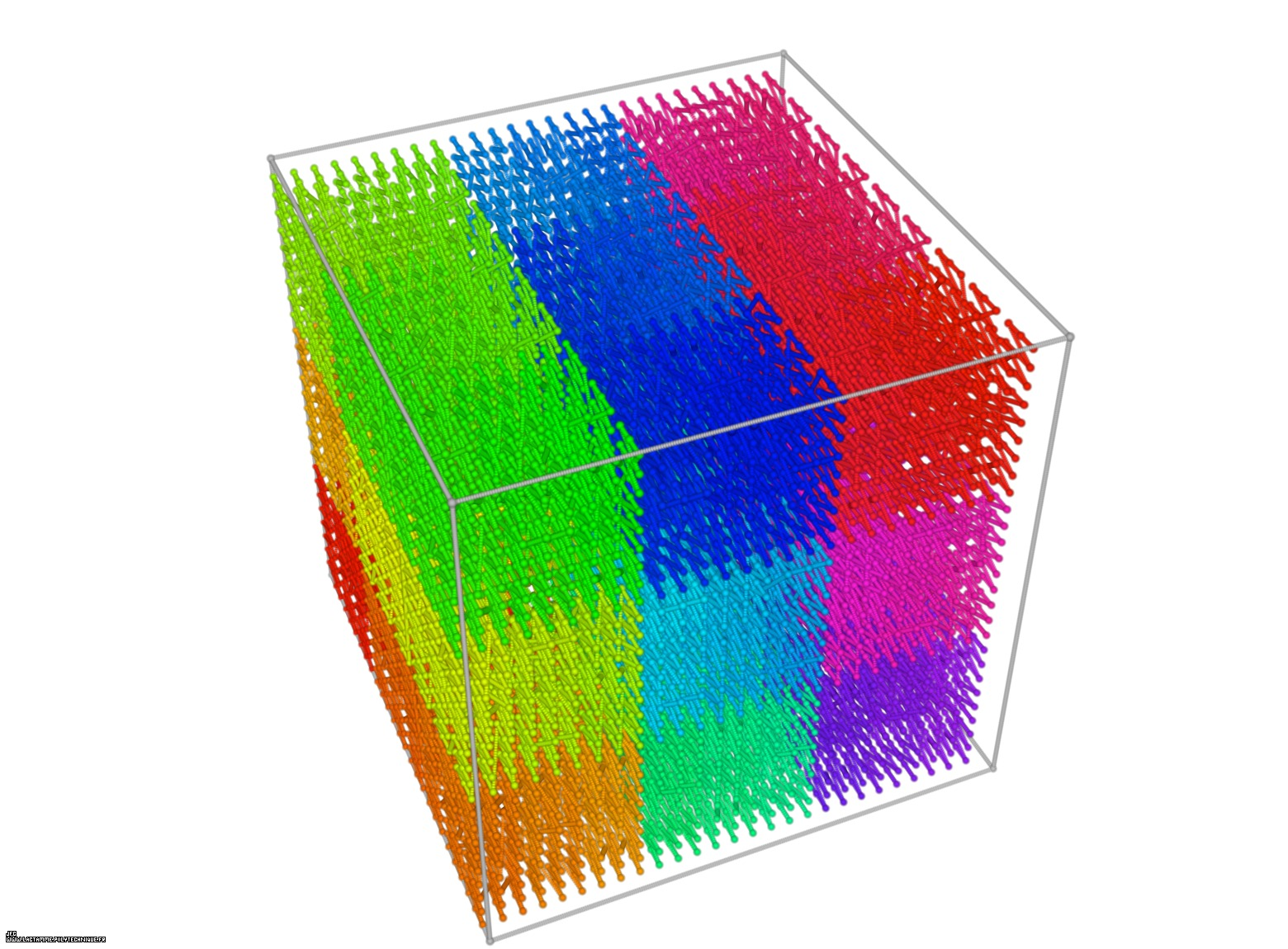The Tridimensional [0,1] --> [0,1]x[0,1]x[0,1] Peano Surjection -T defined with 9 digits- [La surjection tridimensionnelle [0,1] --> [0,1]x[0,1]x[0,1] de Peano -T défini avec 9 décimales-].

# The bidimensional Peano Surjection:

Giuseppe Peano defined the following surjection :
```                    [0,1] --> [0,1]x[0,1]
```
Let's T being a real number defined using the base 3 :
```                    T    = 0.A1A2A3... ∈ [0,1] with Ai ∈ {0,1,2}
```
Let's X(T) and Y(T) being two real functions of T defined as :
```                    X(T) = 0.B1B2B3... ∈ [0,1] with Bi ∈ {0,1,2}
```
```                    Y(T) = 0.C1C2C3... ∈ [0,1] with Ci ∈ {0,1,2}
```
with :
```                    Bn = A2n-1 if A2+A4+...+A2n-0 is even
```
```                    Bn = 2-A2n-1 otherwise
```

```                    Cn = A2n if A1+A3+...+A2n-1 is even
```
```                    Cn = 2-A2n otherwise
```

These two functions X(T) and Y(T) are the coordinates of a point P(T) inside the [0,1]x[0,1] square. The displayed "curve" -as little spheres- is the trajectory of P(T) when T varies from 0 (lower left corner) to 1-epsilon (upper right corner).

Here are the four first bidimensional Peano curves with an increasing number of digits {2,4,6,8}: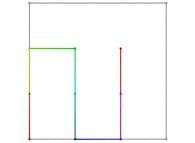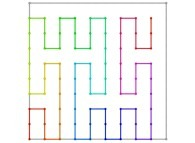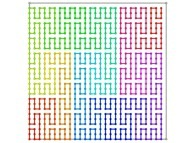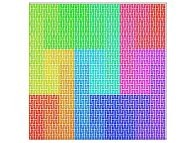[See the used color set to display the parameter T]

# 2-The tridimensional Peano Surjection:

A tridimensional surjection can be defined :
```                    [0,1] --> [0,1]x[0,1]x[0,1]
```
as a generalization of the bidimensional one.

Let's T being a real number defined using the base 3 :
```                    T    = 0.A1A2A3... ∈ [0,1] with Ai ∈ {0,1,2}
```
Let's X(T), Y(T) and Z(T) being three real functions of T defined as:
```                    X(T) = 0.B1B2B3... ∈ [0,1] with Bi ∈ {0,1,2}
```
```                    Y(T) = 0.C1C2C3... ∈ [0,1] with Ci ∈ {0,1,2}
```
```                    Y(T) = 0.D1D2D3... ∈ [0,1] with Di ∈ {0,1,2}
```
with :
```                    Bn = A3n-2 if A3+A6+...+A3n-0 is even
```
```                    Bn = 2-A3n-2 otherwise
```

```                    Cn = A3n-1 if A2+A5+...+A3n-1 is even
```
```                    Cn = 2-A3n-1 otherwise
```

```                    Dn = A3n if A1+A4+...+A3n-2 is even
```
```                    Dn = 2-A3n otherwise
```

These three functions X(T), Y(T) and Z(T) are the coordinates of a point P(T) inside the [0,1]x[0,1]x[0,1] cube. The displayed "curve" is the trajectory of P(T) -displayed as little spheres- when T varies from 0 (lower left corner) to 1-epsilon (upper right corner).

Here are the three first tridimensional Peano curves with an increasing number of digits {3,6,9}: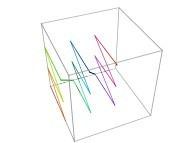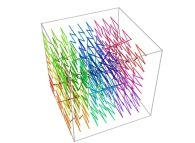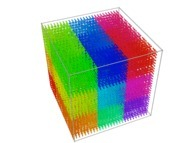[See the used color set to display the parameter T]

See various Tridimensional Hilbert and Peano Curves (possibly including this one):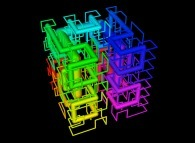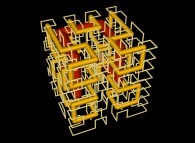See Tridimensional Hilbert Curves, their nodes being "loaded" with some data (related to prime numbers, real number digits,...):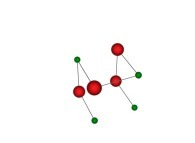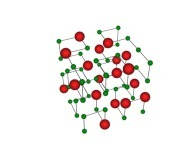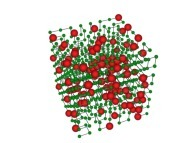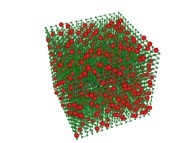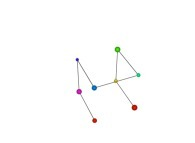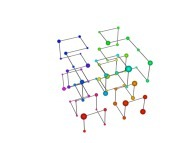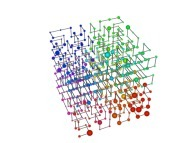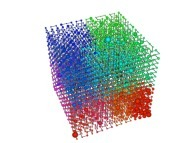See the used color set to display the parameter T.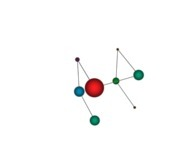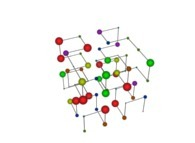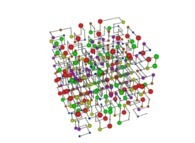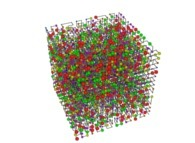See the used color set to display the pi digits.

See various Bidimensional Hilbert and Peano Curves (possibly including this one):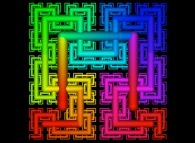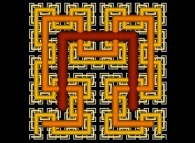See Bidimensional Hilbert Curves, their nodes being "loaded" with some data (related to prime numbers, real number digits,...):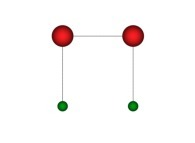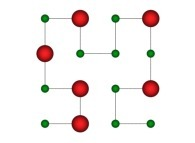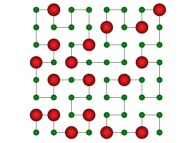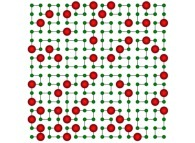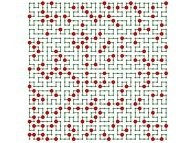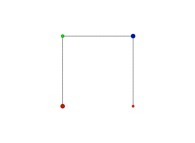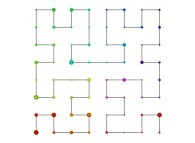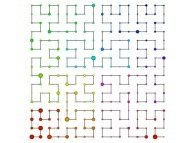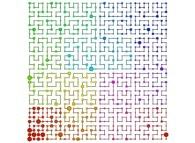See the used color set to display the parameter T.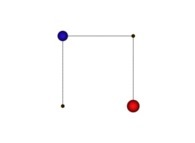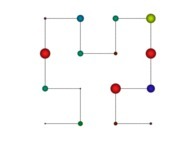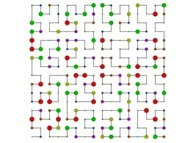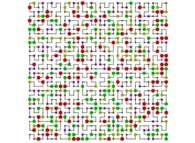See the used color set to display the pi digits.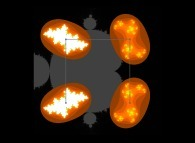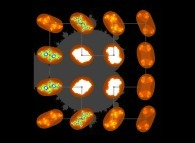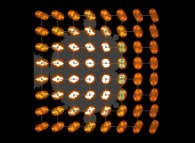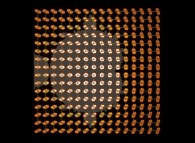(CMAP28 WWW site: this page was created on 04/08/2022 and last updated on 07/04/2023 12:13:25 -CEST-)

[See the generator of this picture [Voir le générateur de cette image]]

[See all related pictures (including this one) [Voir toutes les images associées (incluant celle-ci)]]

[Please visit the related DeterministicFractalGeometry picture gallery [Visitez la galerie d'images DeterministicFractalGeometry associée]]
[Please visit the related NumberTheory picture gallery [Visitez la galerie d'images NumberTheory associée]]

[Go back to AVirtualMachineForExploringSpaceTimeAndBeyond [Retour à AVirtualMachineForExploringSpaceTimeAndBeyond]]

[The Y2K Bug [Le bug de l'an 2000]]

[Site Map, Help and Search [Plan du Site, Aide et Recherche]]
[Mail [Courrier]]
[About Pictures and Animations [A Propos des Images et des Animations]]